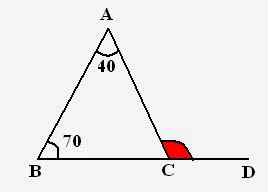# Question# Divisibility Rules: Quantitative Aptitude Section There are some specific rules by which we can determine the divisor of the given number. Today I wil

Polynomial division
ANSWEREDDivisibility Rules: Quantitative Aptitude Section There are some specific rules by which we can determine the divisor of the given number. Today I will discuss divisibility rules from 2 to 19. Using these rules you can easily determine a divisor of given number, however large it may be. Let me tell you the rules of divisibility from 2 to 19.2021-04-16

## Triangles

Triangle = Figure with three sides.  Study the following triangle: A,B,C to points. a, b, c to sides. x, y, z to angles.Perimeter of triangle = a + b + c
Remember that, Sum of all the angles is always $$180^{\circ}$$
i.e. x + y + z = $$180^{\circ}$$

### Classification of Triangles

Basically there are three types of triangles excluding right angle triangle. Let me tell you how they vary from each other.

• Scalene Triangle
• Isosceles Triangle
• Equilateral Triangle

Scalene Triangle: No side of triangle is equal.
Isosceles Triangle: Two sides of triangle are equal.
Equilateral Triangle: All sides of triangle are equal.

 Scalene Isosceles Equilateral Definition a ne b ne c ane b = c a = b = c Area A $$(b/4) \sqrt{4a^2 – b^2}$$ $$(3)^{(1/2)(a^2)/4}$$ Height - $$\sqrt{(4a^2-b^2) /2}$$ $$(3)^{(1/2)a/2}$$

$$A = {s(s-a)(s-b)(s-c)}^{1/2}$$
where, s = (a+ b+ c)/2

### Properties of external angles of Triangle:

1. Sum of all exterior angles is $$360^{\circ}$$
Study the following set of triangles and their exterior angles,a, b, c to Interior angles. p, q, r and s, t, u to Exterior angles.
So, sum of exterior angles = $$360^o$$ i.e. p + q + r = 360^o and s + t + u = $$360^o$$
2. Next property of exterior angle which is important in paper point of view:

External angle = Sum of two internal angles.

For example: In above figures,
r = a + b
q = a + c
s = b + c and so on.

## Right angle Triangle

Following triangle is a right angle triangle i.e. a triangle with one out of three 90^o angle.### Area of right angle triangle

Area = 1/2 times Base times Perpendicular

### Example with Solution

Example: In following figure, CE is perpendicular to AB, angle ACE = 20^o and angle ABD = 50^o. Find angle BDA:Solution: To Find: angle BDA
For this what we need --- angle BAD  Because, Sum of all angles = $$180 ^o$$
Consider, triangle ECA,
CEA + EAC + ACE = $$180^o$$ i.e. $$90^o$$$$20^o$$ + EAC = $$180^o$$ Therefore, EAC = $$70^o$$
Now, come to triangle ABD,
ABD + BDA + BAD = $$180^o$$  $$70^o$$ + $$50^o$$ + BAD = $$180^o$$ Therefore, BAD = $$60^o$$
Example: In given figure. BC is produced to D and angle BAC = $$40^o$$ and angle ABC = $$70^o$$. Find angle ACD:Solution: In above figure, ACD is an exterior angle, and according to property, Exterior angle = Sum of interior angles Therefore, ACD = $$70^o$$ + $$40^o$$ =$$110^o$$
This is not the end of this chapter. These are just the basics. In next session, I will discuss some important results, properties (congruency, similarity) and much more. Always remember, Geometry needs practice and time.